UPDATE October 2020 – Google Sheets now has a randomize range option. I’ve also created a video demonstrating the randomize range option.

## Use the randomize range option

With the randomize range option, it’s a piece of cake to randomize a list of students. Let’s say you have a list of students:

To randomize the range, click on the square to the left of the A, above the 1, to select the entire sheet.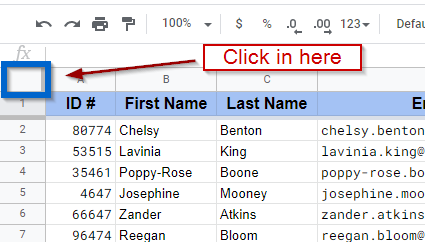Now that the range is selected, go to Data and then Randomize Range: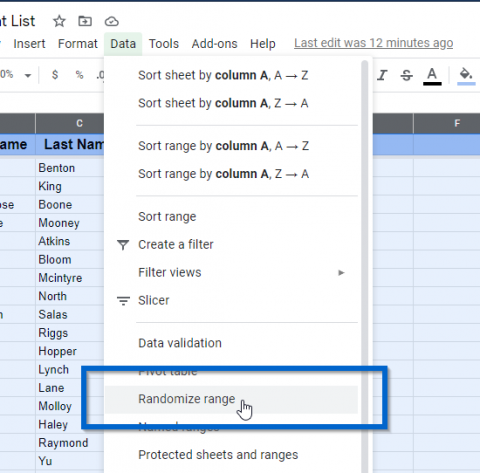Now the students are randomized!

But, Ryan, you say, I want them back to be in alphabetical order!! Yes, you can put them back. Go to Data and select Sort Range.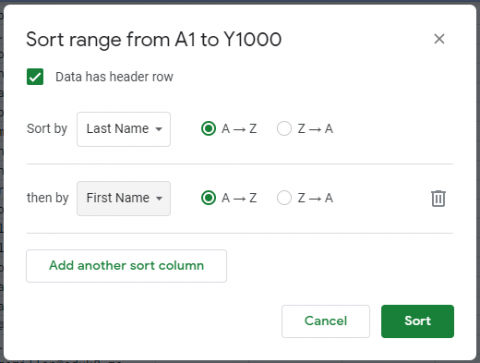Select Data has header row, and then you can sort by Last Name, add another sort column, and then sort by First Name, and your students will be sorted yet again.

The range features, such as randomize range and sort range, work on the selected cells. To make sure you’re sorting what you what to sort, I always select all of the cells… Just to make sure.

## Old way

There are times when you need to randomize students in a list, such as to call on students one at a time or if you want to randomly group students. A quick way to do that is to use Google Sheets. This isn’t limited to Google Sheets, it will also work in other spreadsheet programs.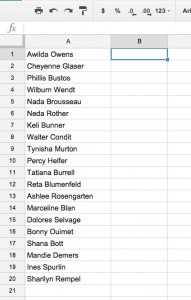Put your list of names in the first column. In the second column, enter the following function:

`=rand()`

Then fill down the second column with that function. In a spreadsheet, formulas start with the equals sign (=). Formulas are used to calculate or assign a different value and store that value in the cell which contains the formula. For this example, we are going to use the rand() function.

A function is a command that will return a value based on its purpose. The rand() function returns a fractional random number between 0 and 1. So our formula in each cell beside the name will be set to a random number between 0 and 1 and will be used to randomize students. There is also the randbetween() function that will return numbers between any two sets of integers, but for our purpose rand() is all we need.

Once that is done, you’re ready to randomize. In the header for the second column, press the triangle menu and select Sort A-Z.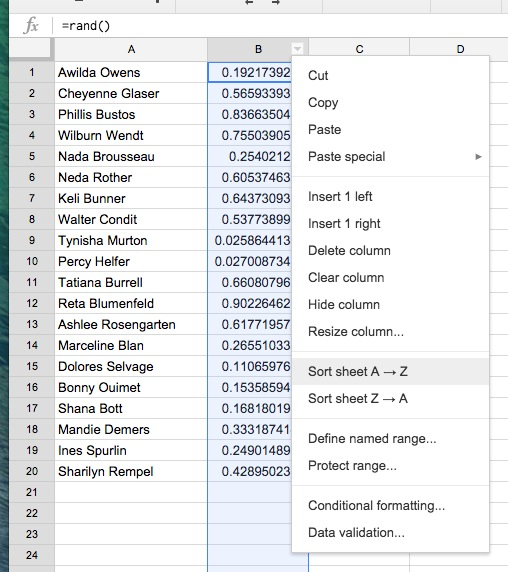Your names are now in random order. Every time you re-sort, your names will be in a different order because the rand() function will return a different random number. Why this works is that Google Sheets will keep the content on each row together.

## Uses for randomized students

You can use the third column to set them in groups (such as putting the students in groups of 4, in the 3 column, put a 1 in the first four spots, a 2 in the next four, and so on). I’m sure there are other ways of doing this, let me know in the comments!Be sure to subscribe to my YouTube channel and join your fellow educators on the Eduk8me email list!